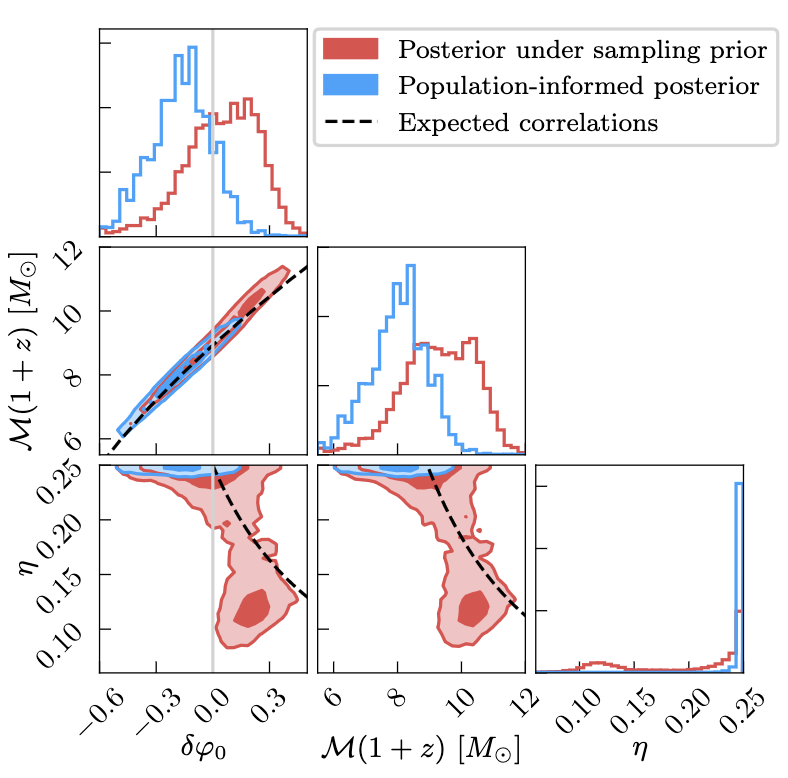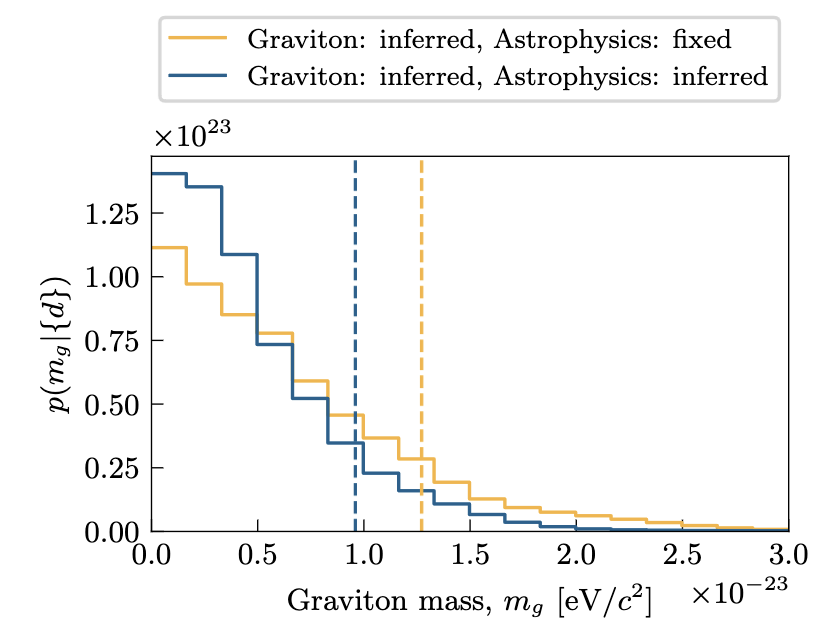# Garbage [priors] in, garbage [posteriors] out: The importance of astrophysics in tests of general relativity

Authors: Ethan Payne, Maximiliano Isi, Katerina Chatziioannou, Will M. Farr

First author’s institution: Department of Physics, California Institute of Technology, Pasadena, California

Status: Preprint, available on arXiv

General Relativity (GR) is our best model of gravity. It just works. So. Well. It passes every test that we apply to it with flying colours. And yet, we know that is incomplete. It doesn’t play well with the Standard Model, our best model of the electromagnetic, strong, and weak forces, and it appears to break down at the singularity of black holes. It also cannot explain dark matter or dark energy. There have been many attempts to measure deviations between an experimental result and its prediction from GR, but so far, no one has been able to find cracks in Einstein’s masterpiece.

When gravitational waves (GWs) were first detected by LIGO in 2015, it provided a new messenger to measure GR-related phenomena. For example, deviations between the predicted and measured waveform of a GW from a stellar-mass black hole binary merger could infer the existence of physics beyond GR. This would be a big breakthrough in fundamental physics.

## Fitting models to data

GW wavelets are fitted to LIGO data using a Bayesian likelihood model – simply, what is the probability of a waveform given the data? The likelihood model relates a set of model parameters with each other, which in this case are parameters that describe deviations from GR and parameters that describe the population of black holes, with data from all of the GW events detected so far. In addition to the likelihood, Bayesian analysis requires a set of priors. The prior of a model parameter is a probability distribution from which we sample, and our choice of prior encapsulates our prior knowledge of the parameter. For example, if we don’t know anything about a parameter beforehand, we set the prior to a uniform distribution, and hence, uninformative. Essentially, we’re saying that the parameter has an equal probability of taking any value between two limits. The product of the prior and likelihood is proportional to the posterior, which is the conditional probability that each parameter has a certain value given your likelihood model and the data. In short, you recover a space of parameter values that the data and model supports the most.

So far, this is how testing GR with LIGO events has been conducted. However, because the parameters describing the population of stellar-mass black hole binaries (such as their spins and masses) are strongly correlated to the set of parameters that describe deviations from GR, poor assumptions on the population of black holes will bias our inference on deviations from GR. For example, the population of primary black holes follows a power-law that decreases with increasing mass. That is significantly more informative than a uniform prior. If you were to use a uniform prior instead, you’re suggesting that there are less low-mass primary black holes in the universe than we know there are! Hence, a uniform prior would recover a biased result towards low-mass black holes.

To demonstrate this problem, Figure 1 compares the posterior distributions of a model with uniform priors with a model including astrophysically-motivated priors. For the same data and likelihood model, the results are strikingly different. The first column is a coefficient that describes deviations from GR – if GR is correct, this parameter should be zero. The other two columns are the posteriors on the detector-frame chirp mass of the merger, and the mass ratio of the merging black holes. Including astrophysical information (blue) shows more of a preference for binaries with a more equal mass ratio than the model with uninformative priors. Hence, a lower merger chirp mass is preferred, as well as a more negative deviation coefficient. In contrast, using uniform priors reaches the opposite conclusion.Figure 1: Posteriors of models with (blue) and without (red) astrophysically-informed priors. Changing the priors recovers significantly different results. (Figure 1 of paper)

## Weighing on a hypothesised particle

To further their argument that astrophysical knowledge should be included in modeling, the authors use LIGO data to recover the mass of the graviton,$m_g$. The graviton is a hypothesised particle that mediates gravitational interactions, equivalent to how the photon mediates electromagnetic interactions. The graviton is expected to be massless, however, some modified theories of GR predict that the graviton has some mass. As such, constraining the mass of the graviton is an important test of GR.

Figure 2 shows the posterior distributions of the recovered mass of the graviton given LIGO’s detections. When using uniform priors on the population of black hole binaries (yellow), the mass of the graviton is constrained to$m_g \leq 1.3 \times 10^{-23} \mathrm{eV}/c^2$ at the 90% confidence level. When an astrophysical prior is applied (blue), the constraint is reduced to$m_g \leq 9.6 \times 10^{-24} \mathrm{eV}/c^2$, and there is more support for a massless graviton ($m_g=0$), and hence less support for deviations from GR.Figure 2: The distribution of inferred graviton mass when the astrophysical prior is (blue) and isn’t (yellow) included. When including this information, the graviton mass is more constrained and shows more support for a massless graviton, and hence shows less support for deviations from GR.

In conclusion, the authors of this paper strongly advocate for astrophysically informative priors when conducting tests on GR, as well as to infer other astrophysical and cosmological properties, and the inference of the equation-of-state of massive, compact objects such as neutron stars. Such considerations will reduce bias from uninformative priors. However, it’s important to note that such priors will not completely erase bias, and care must be taken to properly sample the prior space. I, myself, have succumbed to Neal’s Funnel of Hell far too often as a result of difficulties sampling the prior space… You have been warned.

Edited by Jack Lubin